Скачать презентацию Variation Functions Warm Up Lesson Presentation Lesson Quiz

• Количество слайдов: 40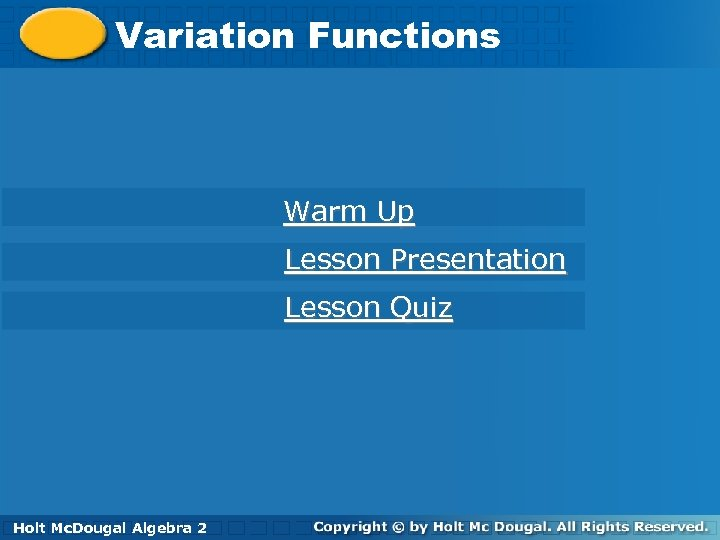Variation Functions Warm Up Lesson Presentation Lesson Quiz Holt Algebra 2 Holt Mc. Dougal Algebra 2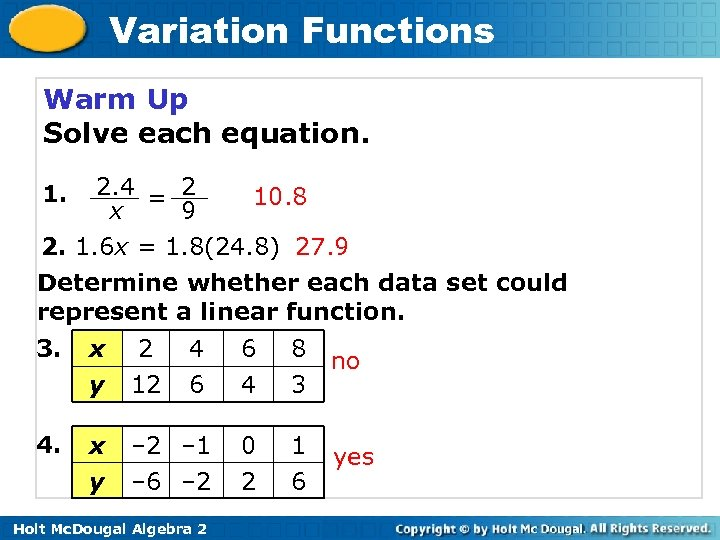Variation Functions Warm Up Solve each equation. 2. 4 = 2 10. 8 x 9 2. 1. 6 x = 1. 8(24. 8) 27. 9 1. Determine whether each data set could represent a linear function. 3. x y 2 12 4 6 4. x y – 2 – 1 – 6 – 2 Holt Mc. Dougal Algebra 2 6 4 8 3 no 0 2 1 6 yes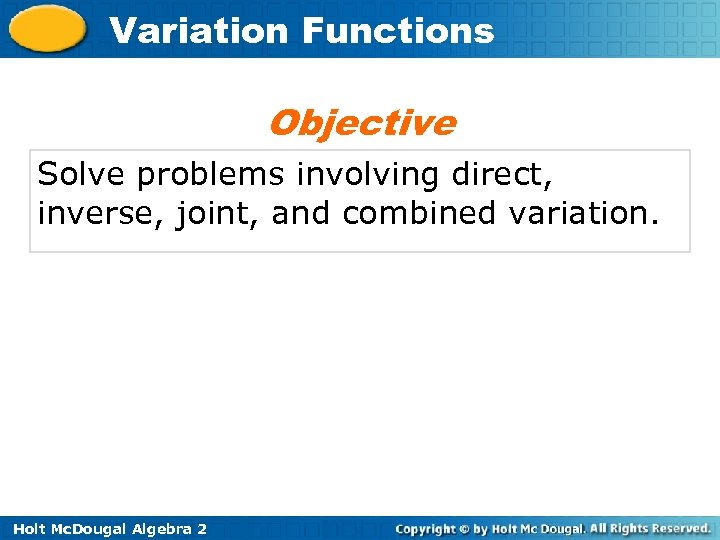Variation Functions Objective Solve problems involving direct, inverse, joint, and combined variation. Holt Mc. Dougal Algebra 2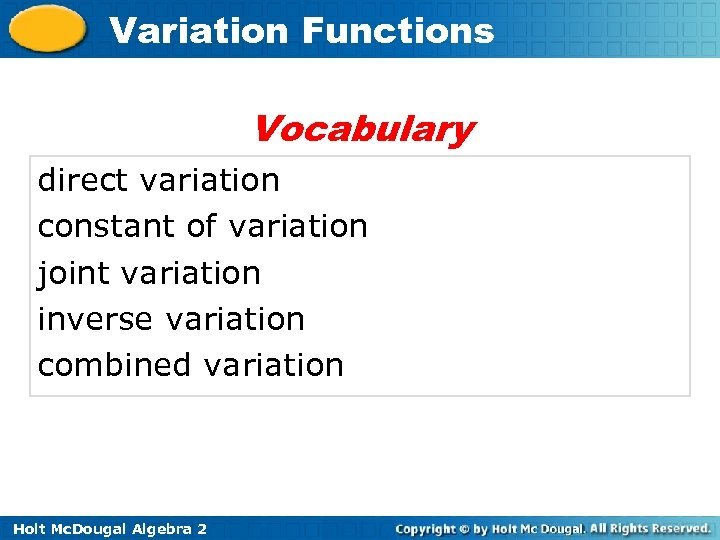Variation Functions Vocabulary direct variation constant of variation joint variation inverse variation combined variation Holt Mc. Dougal Algebra 2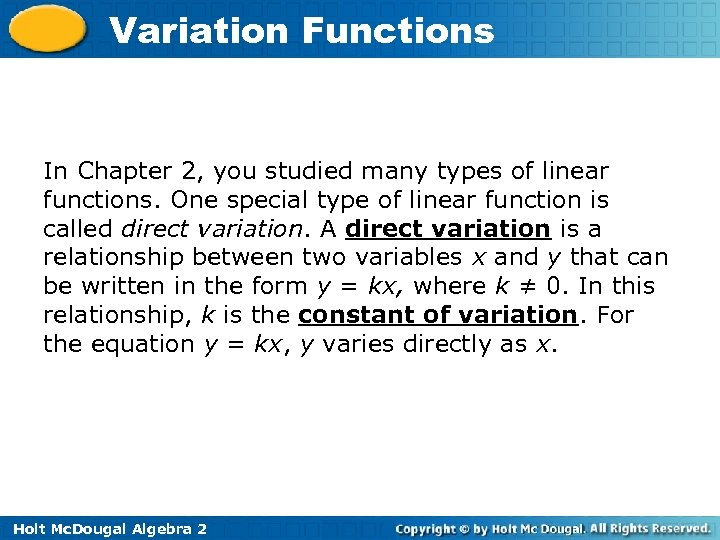Variation Functions In Chapter 2, you studied many types of linear functions. One special type of linear function is called direct variation. A direct variation is a relationship between two variables x and y that can be written in the form y = kx, where k ≠ 0. In this relationship, k is the constant of variation. For the equation y = kx, y varies directly as x. Holt Mc. Dougal Algebra 2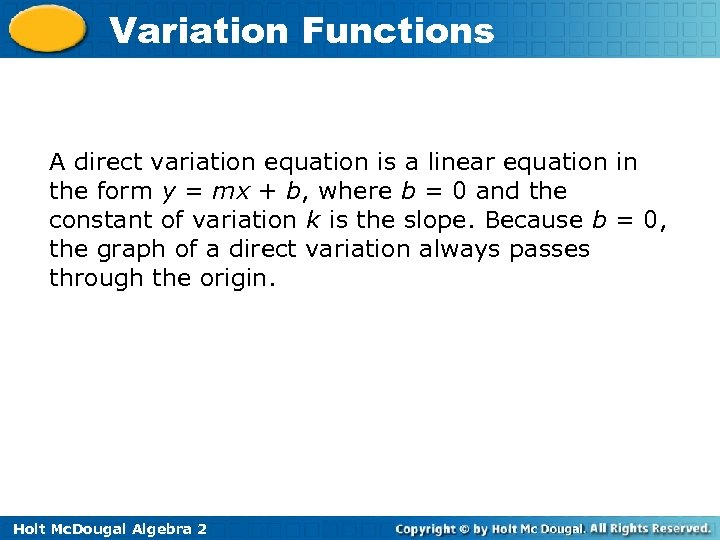Variation Functions A direct variation equation is a linear equation in the form y = mx + b, where b = 0 and the constant of variation k is the slope. Because b = 0, the graph of a direct variation always passes through the origin. Holt Mc. Dougal Algebra 2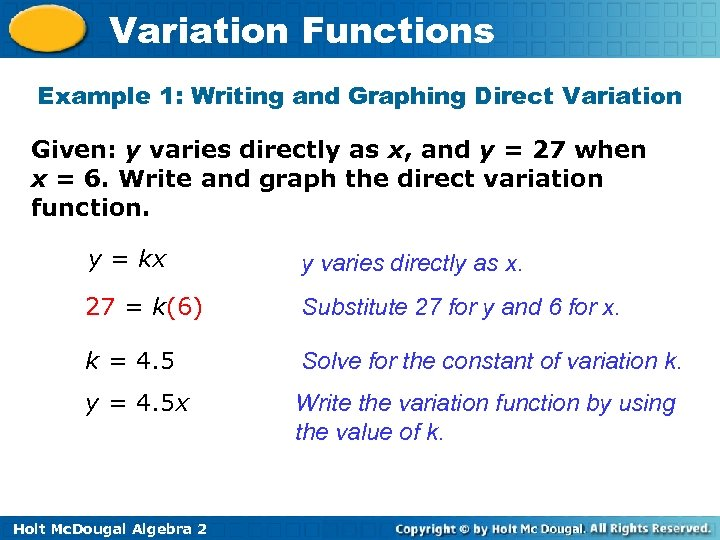Variation Functions Example 1: Writing and Graphing Direct Variation Given: y varies directly as x, and y = 27 when x = 6. Write and graph the direct variation function. y = kx y varies directly as x. 27 = k(6) Substitute 27 for y and 6 for x. k = 4. 5 Solve for the constant of variation k. y = 4. 5 x Write the variation function by using the value of k. Holt Mc. Dougal Algebra 2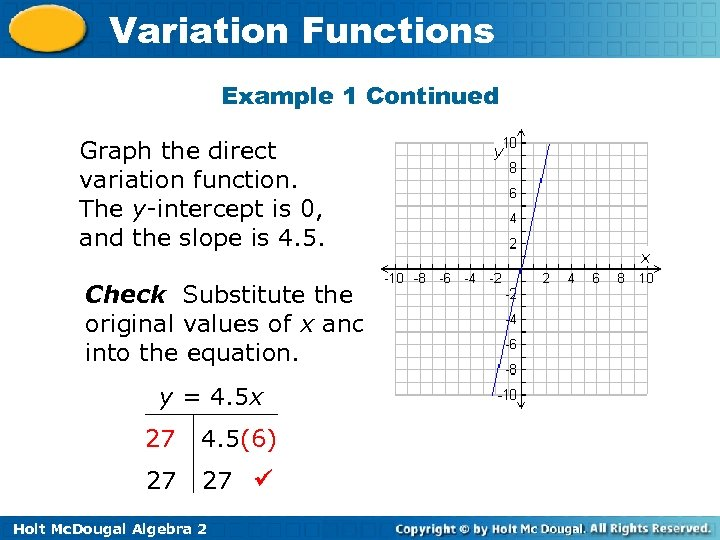Variation Functions Example 1 Continued Graph the direct variation function. The y-intercept is 0, and the slope is 4. 5. Check Substitute the original values of x and y into the equation. y = 4. 5 x 27 4. 5(6) 27 27 Holt Mc. Dougal Algebra 2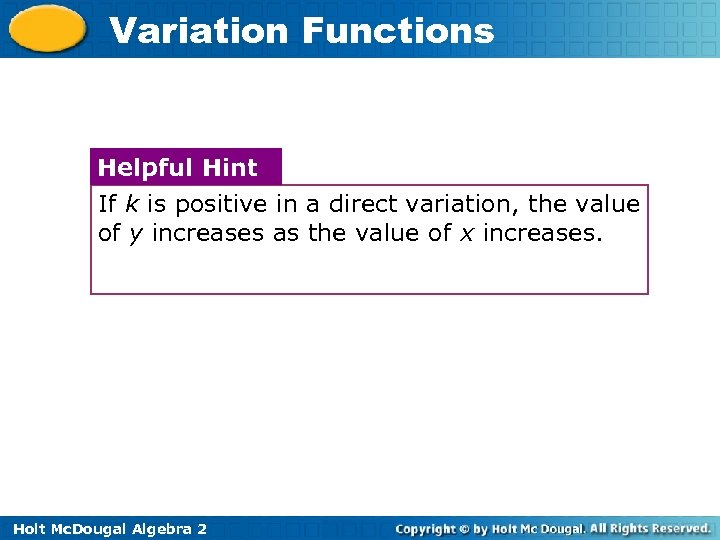Variation Functions Helpful Hint If k is positive in a direct variation, the value of y increases as the value of x increases. Holt Mc. Dougal Algebra 2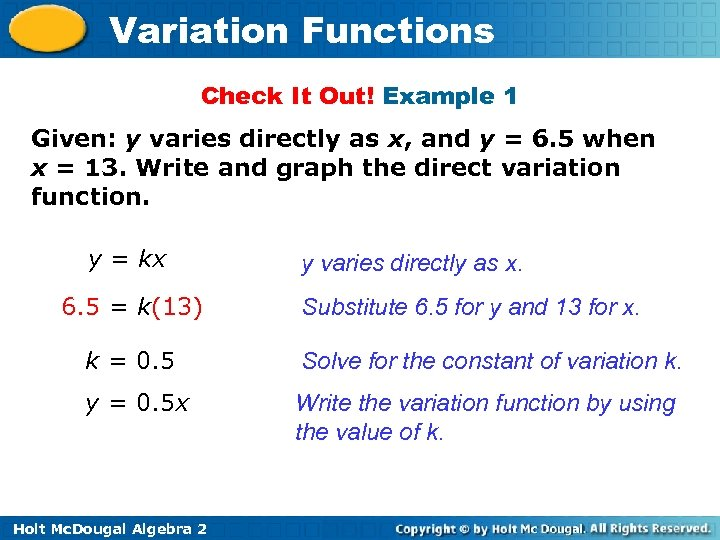Variation Functions Check It Out! Example 1 Given: y varies directly as x, and y = 6. 5 when x = 13. Write and graph the direct variation function. y = kx 6. 5 = k(13) y varies directly as x. Substitute 6. 5 for y and 13 for x. k = 0. 5 Solve for the constant of variation k. y = 0. 5 x Write the variation function by using the value of k. Holt Mc. Dougal Algebra 2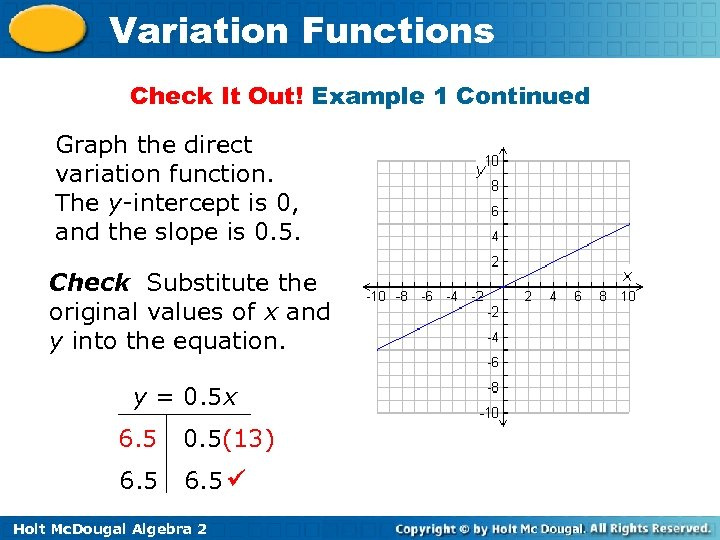Variation Functions Check It Out! Example 1 Continued Graph the direct variation function. The y-intercept is 0, and the slope is 0. 5. Check Substitute the original values of x and y into the equation. y = 0. 5 x 6. 5 0. 5(13) 6. 5 Holt Mc. Dougal Algebra 2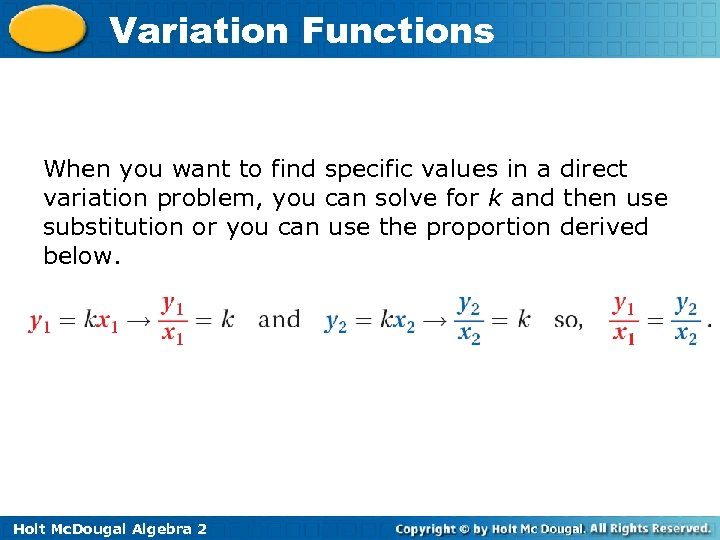Variation Functions When you want to find specific values in a direct variation problem, you can solve for k and then use substitution or you can use the proportion derived below. Holt Mc. Dougal Algebra 2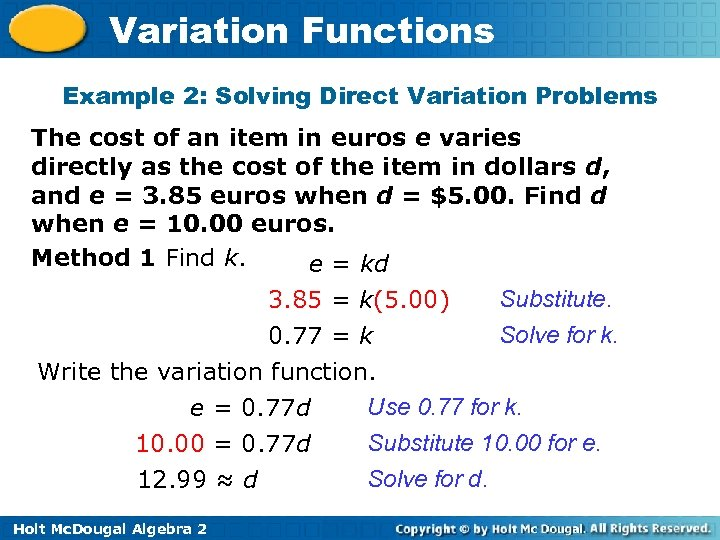Variation Functions Example 2: Solving Direct Variation Problems The cost of an item in euros e varies directly as the cost of the item in dollars d, and e = 3. 85 euros when d = \$5. 00. Find d when e = 10. 00 euros. Method 1 Find k. e = kd Substitute. 3. 85 = k(5. 00) Solve for k. 0. 77 = k Write the variation function. Use 0. 77 for k. e = 0. 77 d Substitute 10. 00 for e. 10. 00 = 0. 77 d Solve for d. 12. 99 ≈ d Holt Mc. Dougal Algebra 2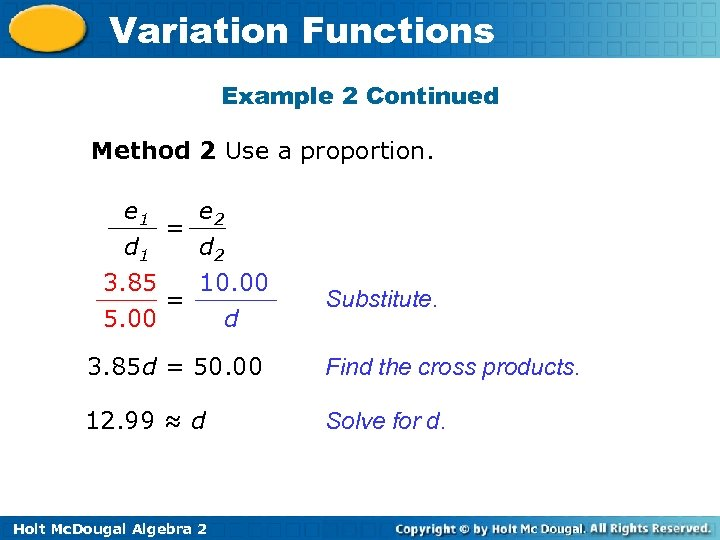Variation Functions Example 2 Continued Method 2 Use a proportion. e 1 e 2 = d 1 d 2 3. 85 10. 00 = 5. 00 d Substitute. 3. 85 d = 50. 00 Find the cross products. 12. 99 ≈ d Solve for d. Holt Mc. Dougal Algebra 2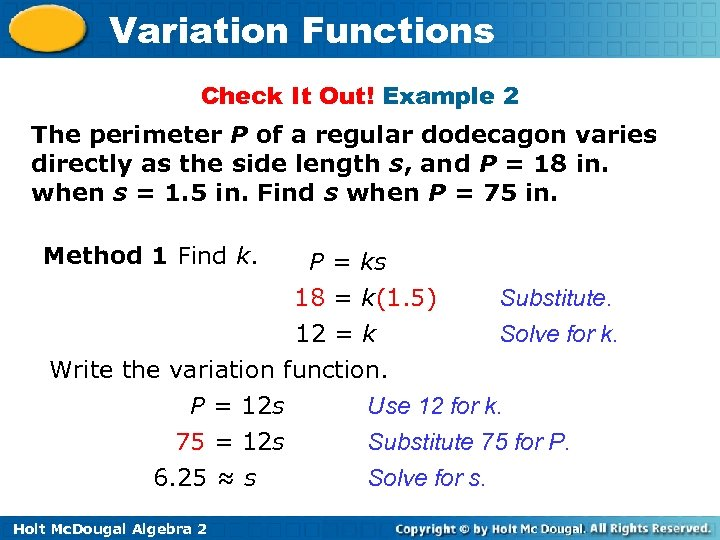Variation Functions Check It Out! Example 2 The perimeter P of a regular dodecagon varies directly as the side length s, and P = 18 in. when s = 1. 5 in. Find s when P = 75 in. Method 1 Find k. P = ks Substitute. 18 = k(1. 5) Solve for k. 12 = k Write the variation function. Use 12 for k. P = 12 s Substitute 75 for P. 75 = 12 s Solve for s. 6. 25 ≈ s Holt Mc. Dougal Algebra 2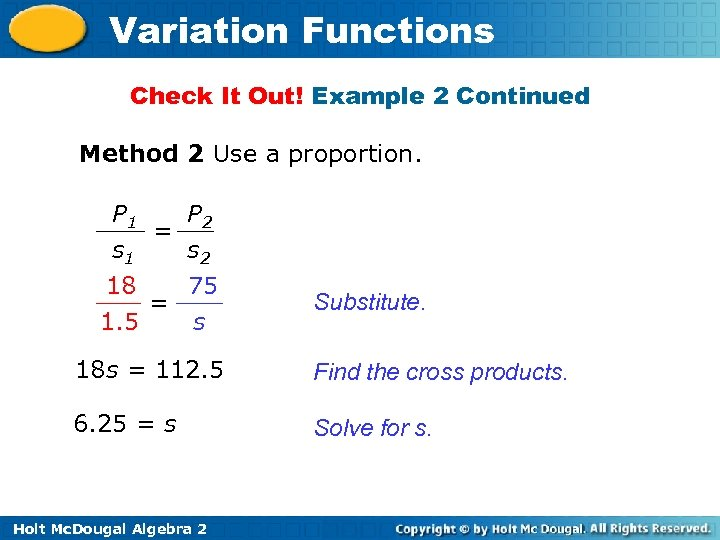Variation Functions Check It Out! Example 2 Continued Method 2 Use a proportion. P 1 P 2 = s 1 s 2 18 75 = 1. 5 s Substitute. 18 s = 112. 5 Find the cross products. 6. 25 = s Solve for s. Holt Mc. Dougal Algebra 2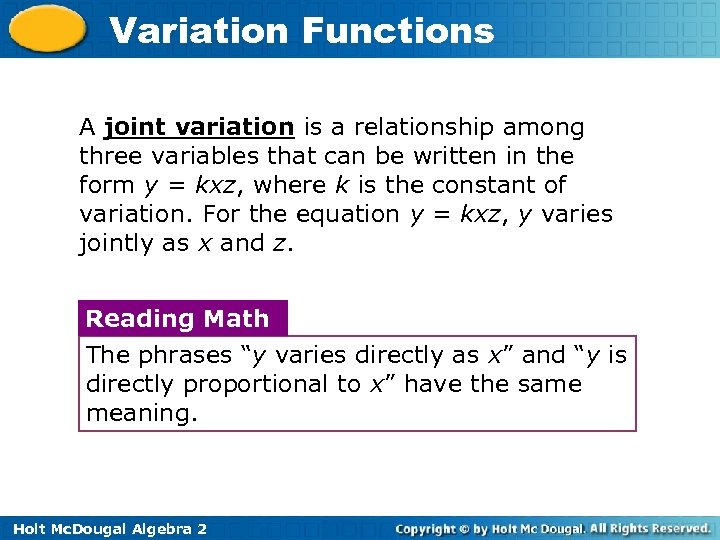Variation Functions A joint variation is a relationship among three variables that can be written in the form y = kxz, where k is the constant of variation. For the equation y = kxz, y varies jointly as x and z. Reading Math The phrases “y varies directly as x” and “y is directly proportional to x” have the same meaning. Holt Mc. Dougal Algebra 2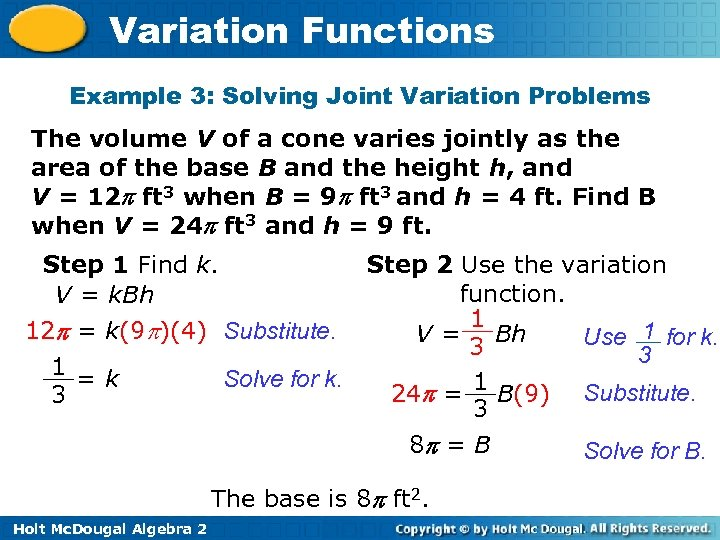Variation Functions Example 3: Solving Joint Variation Problems The volume V of a cone varies jointly as the area of the base B and the height h, and V = 12 ft 3 when B = 9 ft 3 and h = 4 ft. Find B when V = 24 ft 3 and h = 9 ft. Step 1 Find k. V = k. Bh 12 = k(9 )(4) Substitute. 1 =k Solve for k. 3 Step 2 Use the variation function. 1 V = Bh Use 1 for k. 3 3 1 24 = B(9) Substitute. 3 8 = B The base is 8 ft 2. Holt Mc. Dougal Algebra 2 Solve for B.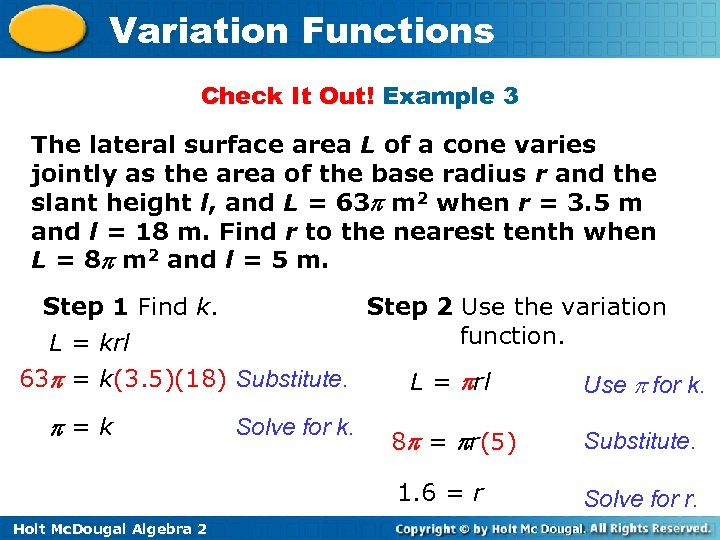Variation Functions Check It Out! Example 3 The lateral surface area L of a cone varies jointly as the area of the base radius r and the slant height l, and L = 63 m 2 when r = 3. 5 m and l = 18 m. Find r to the nearest tenth when L = 8 m 2 and l = 5 m. Step 1 Find k. L = krl Step 2 Use the variation function. 63 = k(3. 5)(18) Substitute. Holt Mc. Dougal Algebra 2 Solve for k. Use for k. 8 = r(5) Substitute. 1. 6 = r =k L = rl Solve for r.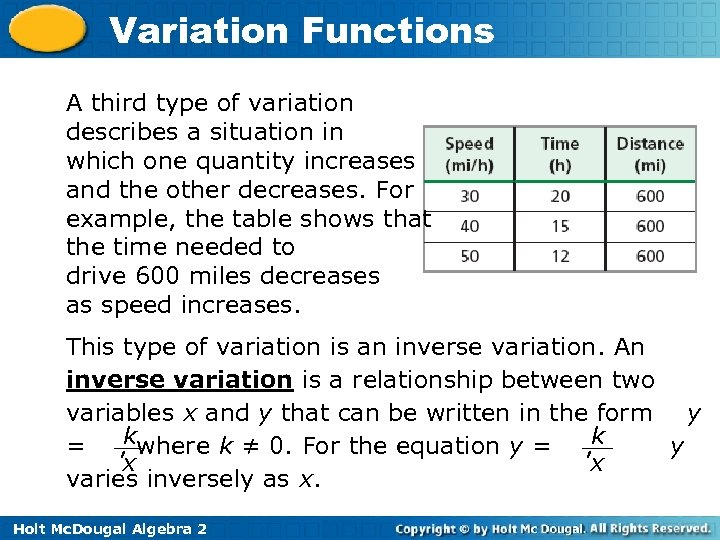Variation Functions A third type of variation describes a situation in which one quantity increases and the other decreases. For example, the table shows that the time needed to drive 600 miles decreases as speed increases. This type of variation is an inverse variation. An inverse variation is a relationship between two variables x and y that can be written in the form y k = , where k ≠ 0. For the equation y = , k y x x varies inversely as x. Holt Mc. Dougal Algebra 2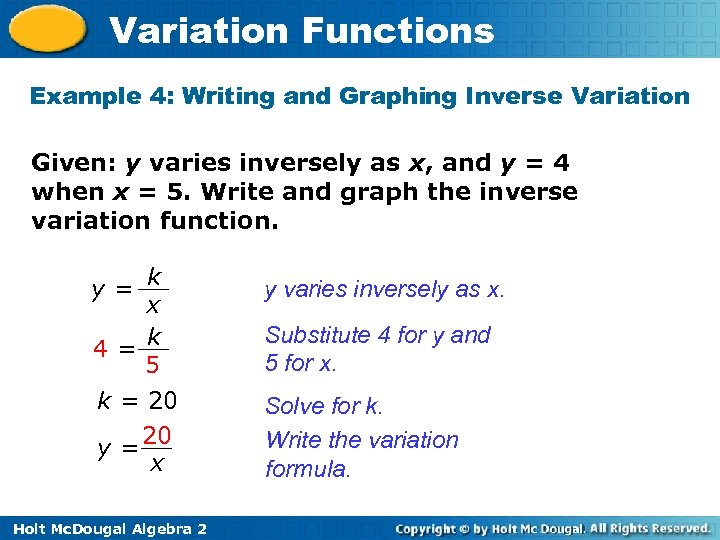Variation Functions Example 4: Writing and Graphing Inverse Variation Given: y varies inversely as x, and y = 4 when x = 5. Write and graph the inverse variation function. y= k x 4= k 5 k = 20 y = 20 x Holt Mc. Dougal Algebra 2 y varies inversely as x. Substitute 4 for y and 5 for x. Solve for k. Write the variation formula.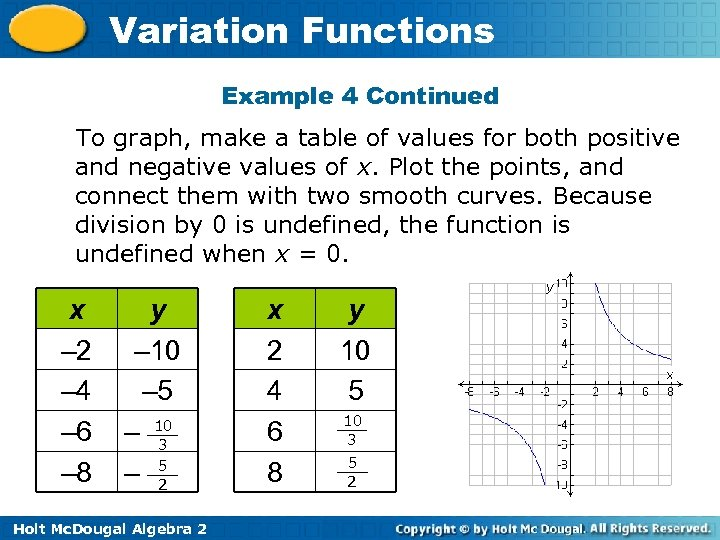Variation Functions Example 4 Continued To graph, make a table of values for both positive and negative values of x. Plot the points, and connect them with two smooth curves. Because division by 0 is undefined, the function is undefined when x = 0. x – 2 – 4 – 6 – 8 y – 10 – 5 – 10 3 5 – 2 Holt Mc. Dougal Algebra 2 x 2 4 6 8 y 10 5 10 3 5 2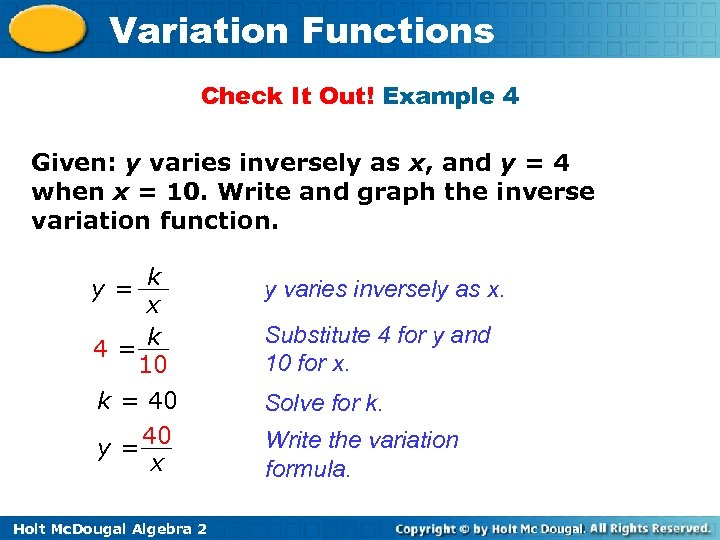Variation Functions Check It Out! Example 4 Given: y varies inversely as x, and y = 4 when x = 10. Write and graph the inverse variation function. y= k x 4= k 10 k = 40 y = 40 x Holt Mc. Dougal Algebra 2 y varies inversely as x. Substitute 4 for y and 10 for x. Solve for k. Write the variation formula.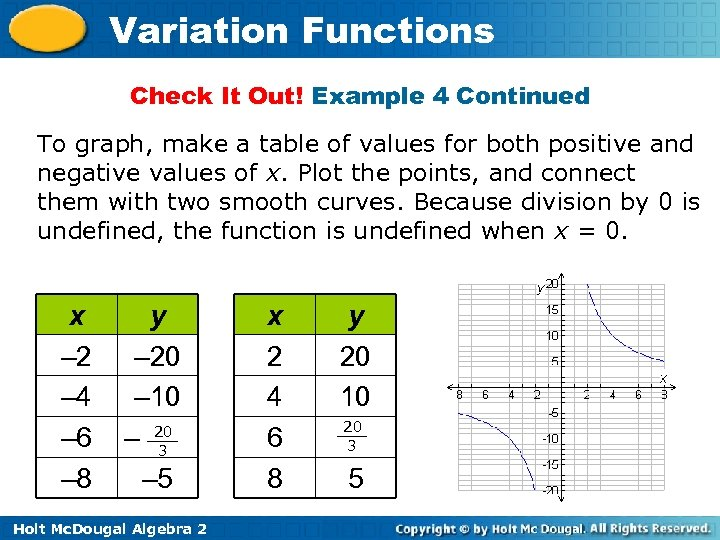Variation Functions Check It Out! Example 4 Continued To graph, make a table of values for both positive and negative values of x. Plot the points, and connect them with two smooth curves. Because division by 0 is undefined, the function is undefined when x = 0. x – 2 – 4 – 6 – 8 y – 20 – 10 – 20 3 – 5 Holt Mc. Dougal Algebra 2 x 2 4 6 8 y 20 10 20 3 5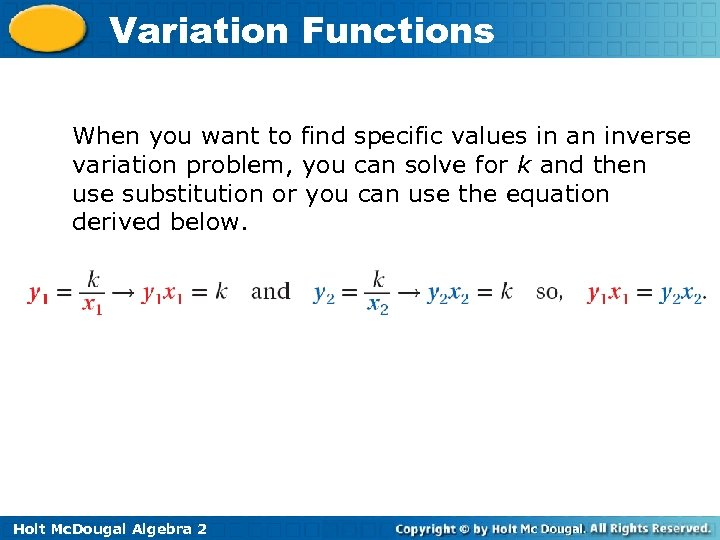Variation Functions When you want to find specific values in an inverse variation problem, you can solve for k and then use substitution or you can use the equation derived below. Holt Mc. Dougal Algebra 2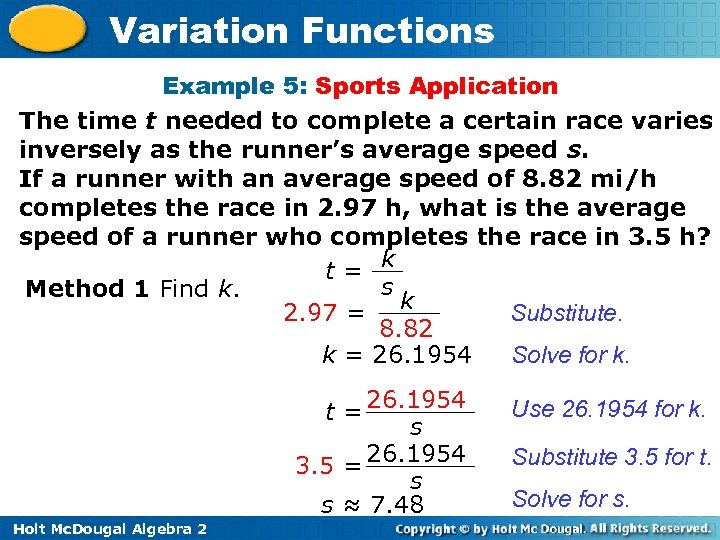Variation Functions Example 5: Sports Application The time t needed to complete a certain race varies inversely as the runner’s average speed s. If a runner with an average speed of 8. 82 mi/h completes the race in 2. 97 h, what is the average speed of a runner who completes the race in 3. 5 h? t= k s Method 1 Find k. Substitute. 2. 97 = k 8. 82 Solve for k. k = 26. 1954 t = 26. 1954 s 3. 5 = 26. 1954 s s ≈ 7. 48 Holt Mc. Dougal Algebra 2 Use 26. 1954 for k. Substitute 3. 5 for t. Solve for s.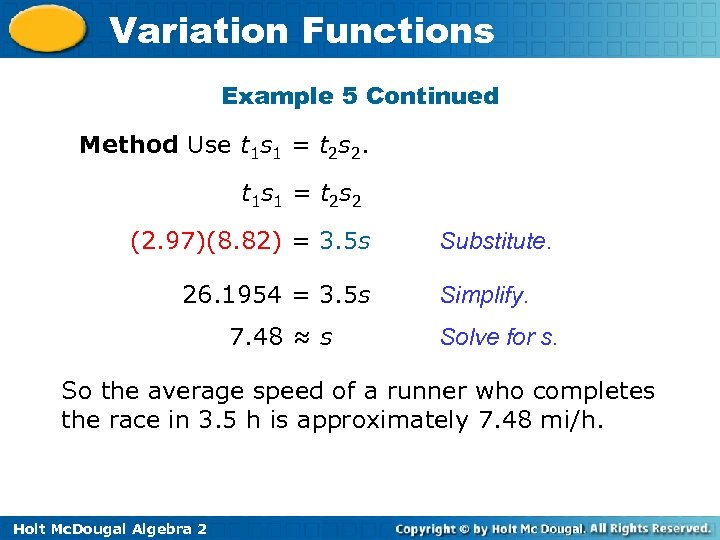Variation Functions Example 5 Continued Method Use t 1 s 1 = t 2 s 2. t 1 s 1 = t 2 s 2 (2. 97)(8. 82) = 3. 5 s 26. 1954 = 3. 5 s 7. 48 ≈ s Substitute. Simplify. Solve for s. So the average speed of a runner who completes the race in 3. 5 h is approximately 7. 48 mi/h. Holt Mc. Dougal Algebra 2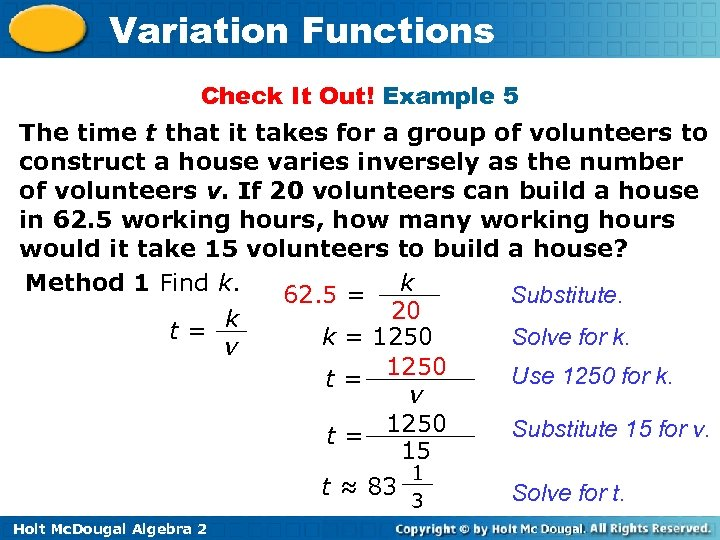Variation Functions Check It Out! Example 5 The time t that it takes for a group of volunteers to construct a house varies inversely as the number of volunteers v. If 20 volunteers can build a house in 62. 5 working hours, how many working hours would it take 15 volunteers to build a house? Method 1 Find k. Substitute. 62. 5 = k 20 k t= Solve for k. k = 1250 v Use 1250 for k. t = 1250 v Substitute 15 for v. t = 1250 15 t ≈ 83 Holt Mc. Dougal Algebra 2 1 3 Solve for t.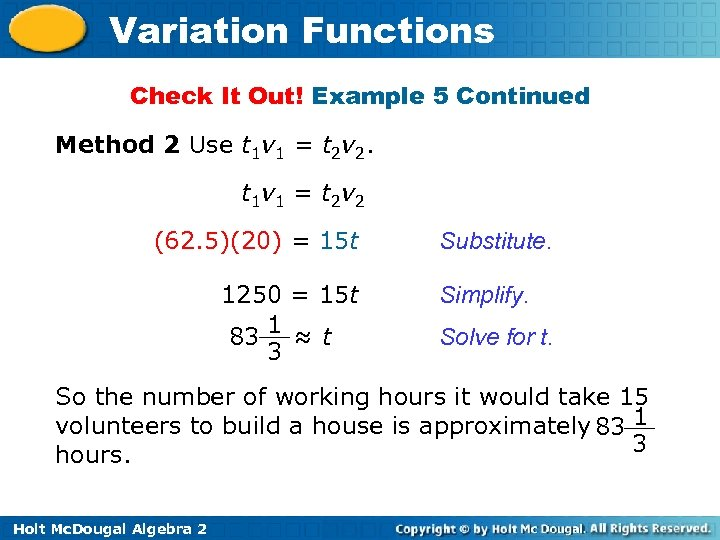Variation Functions Check It Out! Example 5 Continued Method 2 Use t 1 v 1 = t 2 v 2. t 1 v 1 = t 2 v 2 (62. 5)(20) = 15 t 1250 = 15 t 1 83 ≈ t 3 Substitute. Simplify. Solve for t. So the number of working hours it would take 15 volunteers to build a house is approximately 83 1 3 hours. Holt Mc. Dougal Algebra 2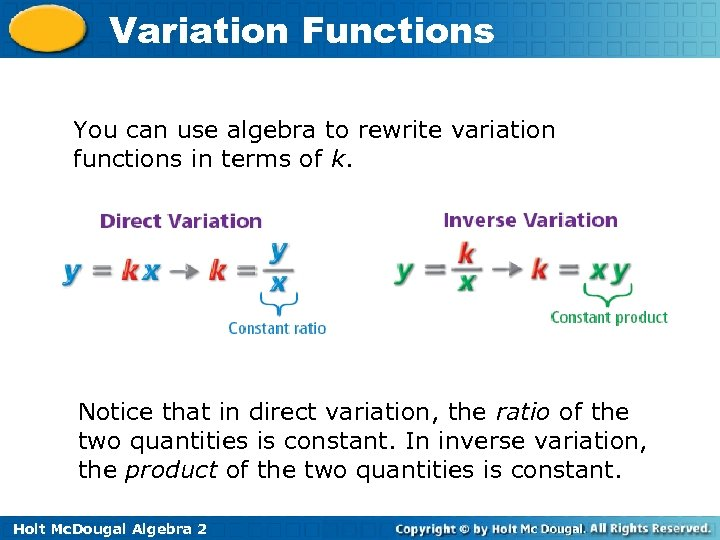Variation Functions You can use algebra to rewrite variation functions in terms of k. Notice that in direct variation, the ratio of the two quantities is constant. In inverse variation, the product of the two quantities is constant. Holt Mc. Dougal Algebra 2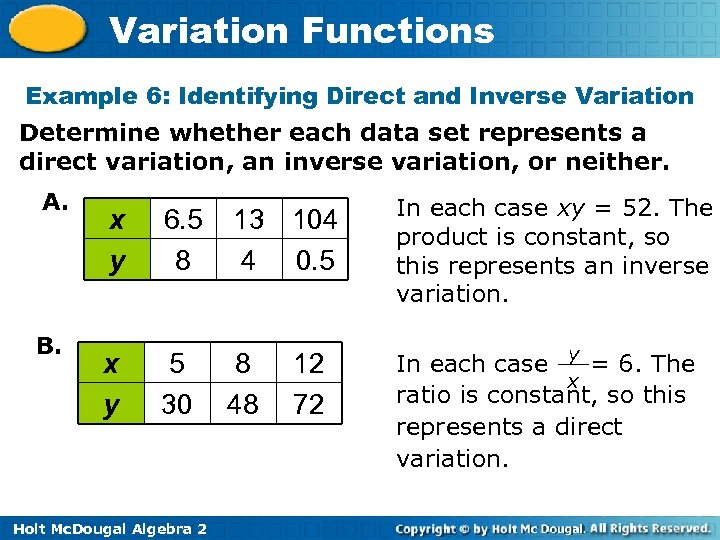Variation Functions Example 6: Identifying Direct and Inverse Variation Determine whether each data set represents a direct variation, an inverse variation, or neither. A. B. x y 6. 5 8 13 104 4 0. 5 In each case xy = 52. The product is constant, so this represents an inverse variation. x y 5 30 8 48 In each case y = 6. The x ratio is constant, so this represents a direct variation. Holt Mc. Dougal Algebra 2 12 72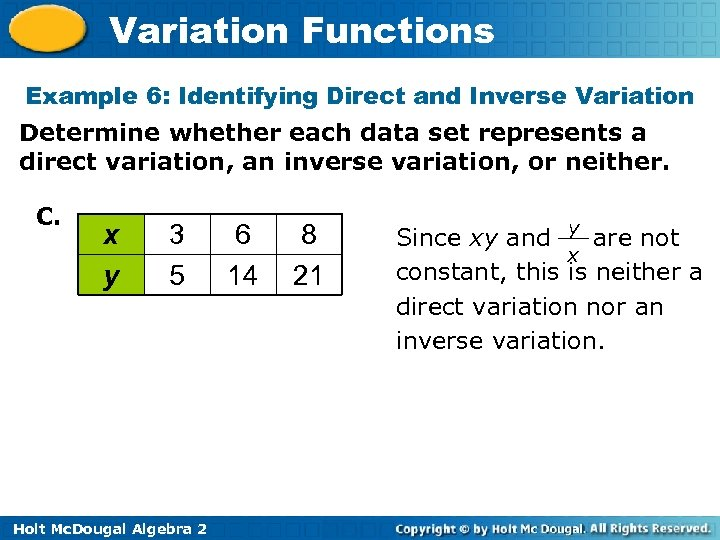Variation Functions Example 6: Identifying Direct and Inverse Variation Determine whether each data set represents a direct variation, an inverse variation, or neither. C. x y 3 5 Holt Mc. Dougal Algebra 2 6 14 8 21 Since xy and y are not x constant, this is neither a direct variation nor an inverse variation.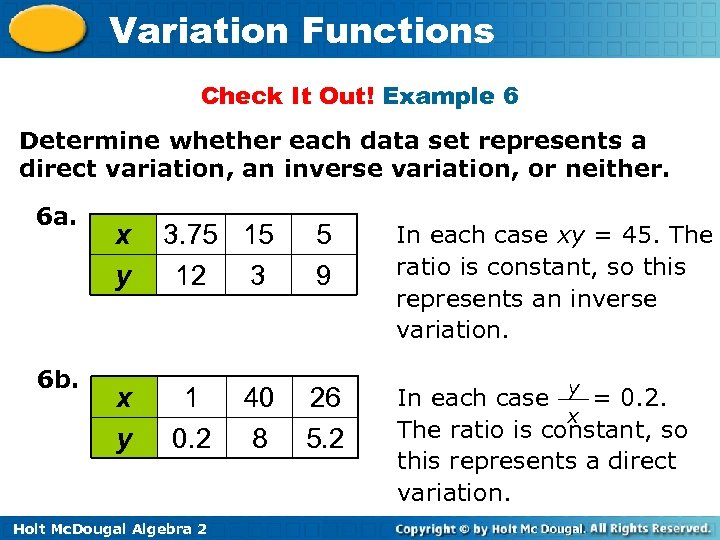Variation Functions Check It Out! Example 6 Determine whether each data set represents a direct variation, an inverse variation, or neither. 6 a. 6 b. x y 3. 75 15 12 3 1 0. 2 Holt Mc. Dougal Algebra 2 40 8 5 9 26 5. 2 In each case xy = 45. The ratio is constant, so this represents an inverse variation. In each case y = 0. 2. x The ratio is constant, so this represents a direct variation.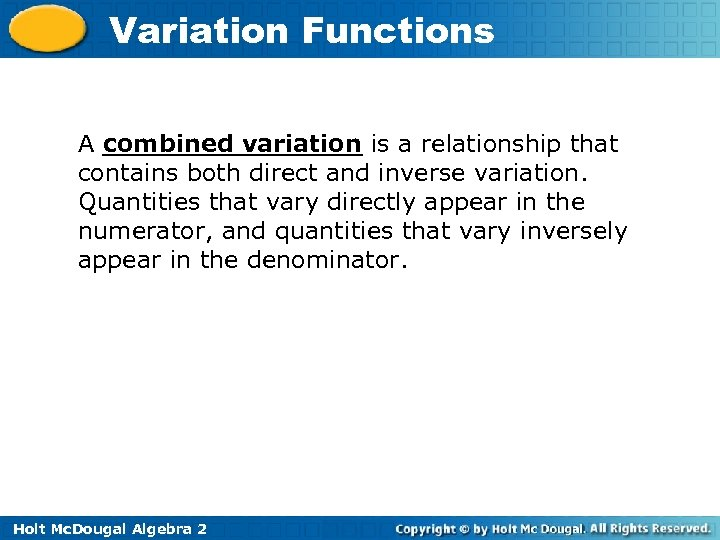Variation Functions A combined variation is a relationship that contains both direct and inverse variation. Quantities that vary directly appear in the numerator, and quantities that vary inversely appear in the denominator. Holt Mc. Dougal Algebra 2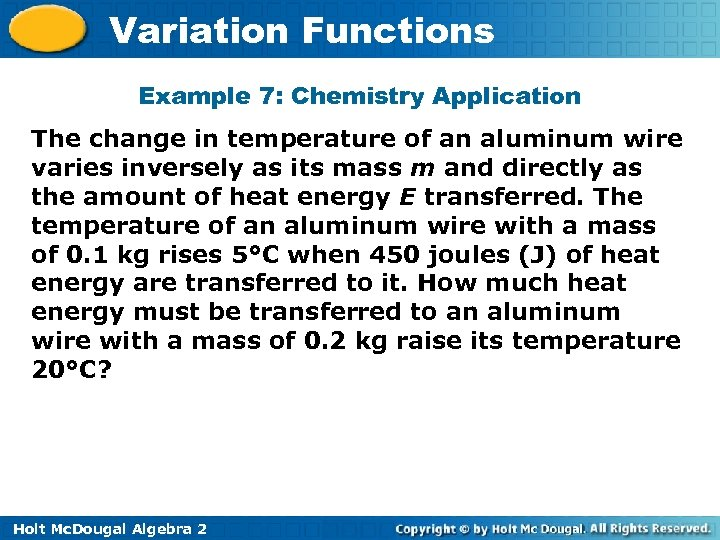Variation Functions Example 7: Chemistry Application The change in temperature of an aluminum wire varies inversely as its mass m and directly as the amount of heat energy E transferred. The temperature of an aluminum wire with a mass of 0. 1 kg rises 5°C when 450 joules (J) of heat energy are transferred to it. How much heat energy must be transferred to an aluminum wire with a mass of 0. 2 kg raise its temperature 20°C? Holt Mc. Dougal Algebra 2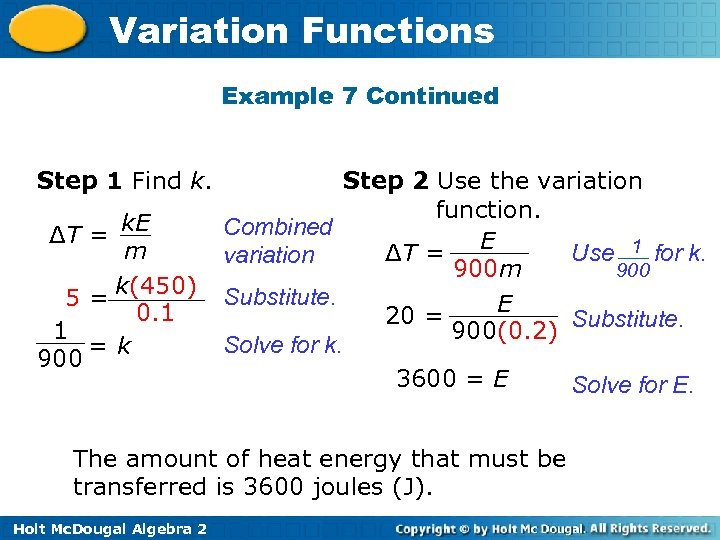Variation Functions Example 7 Continued Step 1 Find k. ΔT = k. E m 5 = k(450) 0. 1 1 =k 900 Step 2 Use the variation function. Combined Use 1 for k. ΔT = E variation 900 m 900 Substitute. E 20 = Substitute. 900(0. 2) Solve for k. 3600 = E Solve for E. The amount of heat energy that must be transferred is 3600 joules (J). Holt Mc. Dougal Algebra 2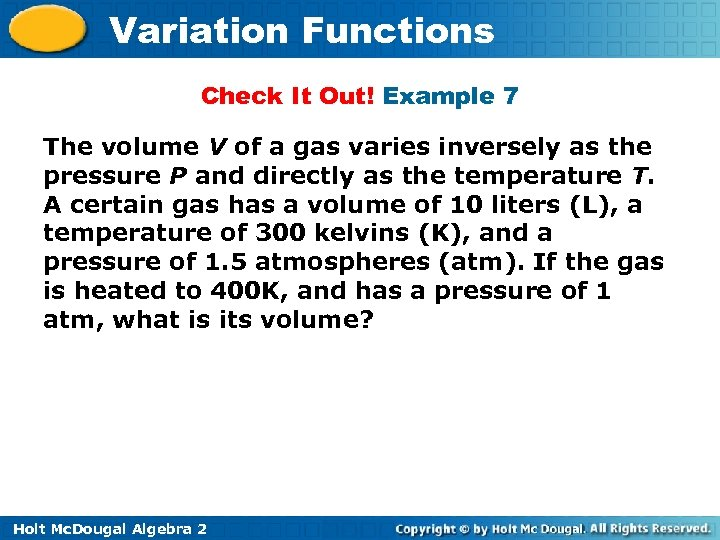Variation Functions Check It Out! Example 7 The volume V of a gas varies inversely as the pressure P and directly as the temperature T. A certain gas has a volume of 10 liters (L), a temperature of 300 kelvins (K), and a pressure of 1. 5 atmospheres (atm). If the gas is heated to 400 K, and has a pressure of 1 atm, what is its volume? Holt Mc. Dougal Algebra 2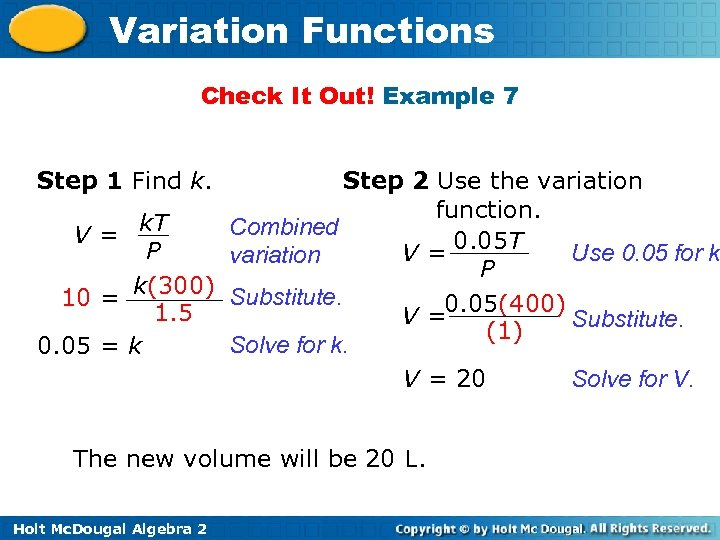Variation Functions Check It Out! Example 7 Step 1 Find k. Step 2 Use the variation function. k. T Combined V= P Use 0. 05 for k V = 0. 05 T variation P 10 = k(300) Substitute. 1. 5 V =0. 05(400) Substitute. (1) Solve for k. 0. 05 = k Solve for V. V = 20 The new volume will be 20 L. Holt Mc. Dougal Algebra 2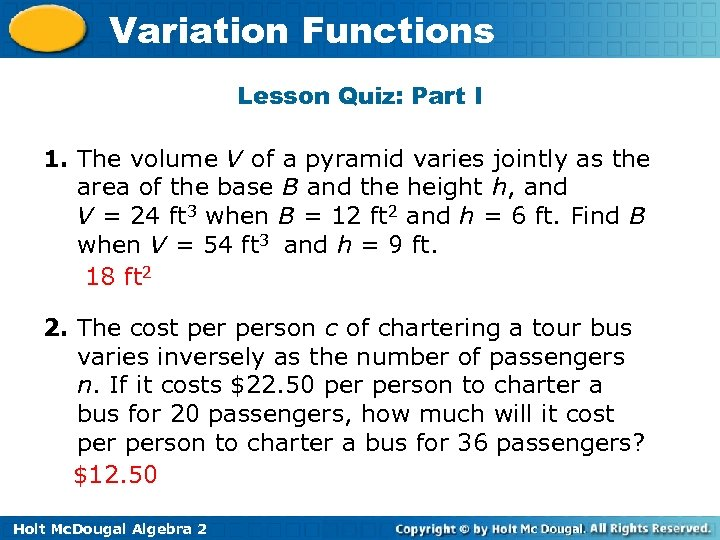Variation Functions Lesson Quiz: Part I 1. The volume V of a pyramid varies jointly as the area of the base B and the height h, and V = 24 ft 3 when B = 12 ft 2 and h = 6 ft. Find B when V = 54 ft 3 and h = 9 ft. 18 ft 2 2. The cost person c of chartering a tour bus varies inversely as the number of passengers n. If it costs \$22. 50 person to charter a bus for 20 passengers, how much will it cost person to charter a bus for 36 passengers? \$12. 50 Holt Mc. Dougal Algebra 2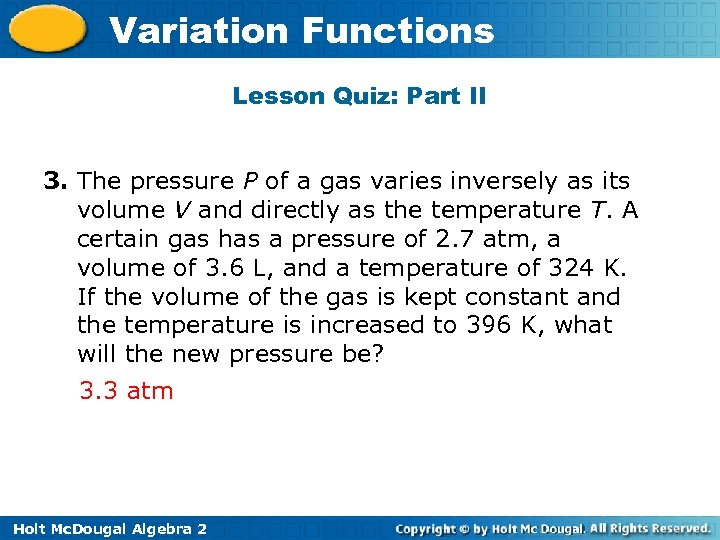Variation Functions Lesson Quiz: Part II 3. The pressure P of a gas varies inversely as its volume V and directly as the temperature T. A certain gas has a pressure of 2. 7 atm, a volume of 3. 6 L, and a temperature of 324 K. If the volume of the gas is kept constant and the temperature is increased to 396 K, what will the new pressure be? 3. 3 atm Holt Mc. Dougal Algebra 2